# Some More Written Practice Questions

Question.1

What is meant by solenoid? How does a current carrying solenoid behave? Give its main use.

Solenoid: A coil of many circular turns of insulated copper wire wound on a cylindrical insulating body (i.e., cardboard etc.) such that its length is greater than its diameter is called solenoid.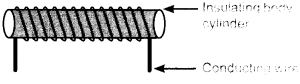When current is flowing through the solenoid, the magnetic field line pattern resembles exactly with those of a bar magnet with the fixed polarity,
i.e. North and South pole at its ends and it acquires the directive and attractive properties similar to bar magnet. Hence, the current carrying solenoid behave as a bar magnet.

Use of current carrying solenoid: It is used to form a temporary magnet called electromagnet as well as permanent magnet.

Question.2

For the current carrying solenoid as shown below, draw magnetic field lines and giving reason explain that out of the three points A, B and C at which point the field strength is maximum and at which point it is minimum.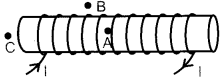Answer.  Outside the solenoid magnetic field is minimum. At the ends of solenoid, magnetic field strength is half to that inside it. So Minimum – at point B; Maximum – at point A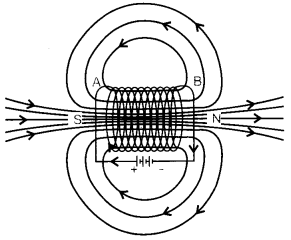Question.3

.List in tabular form two major differences between an electric motor and a
generator.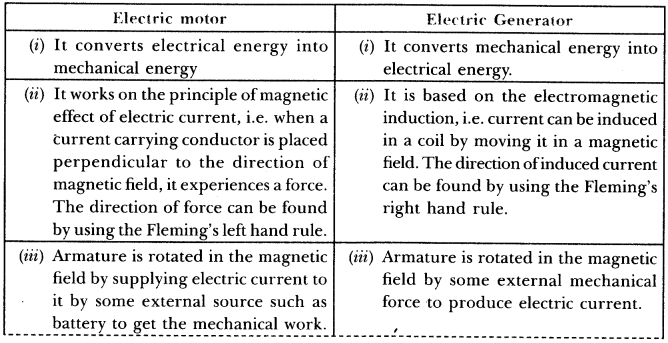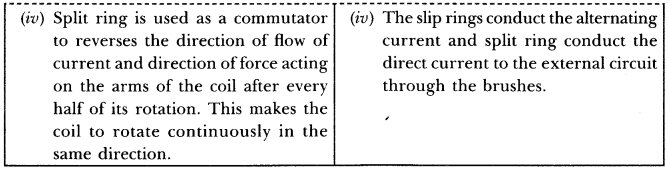Question.4

Two coils C1 and C2are wrapped around a non conducting cylinder. Coil  C1is connected to a battery and key and C2 with galvanometer G. On pressing the key (K), current starts flowing in the coil  C1State your observation in the galvanometer: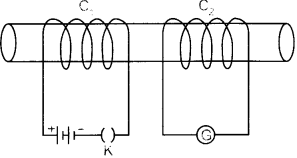(i) When key K is pressed on
(ii) When current in the coil C1 is switched off.
(iii) When the current is passed continuously through coil  C1
(iv) Name and state the phenomenon responsible for the above observation. Write the name of the rule that is used to determine the direction of current produced in the phenomena.

(i) When key is pressed on, the galvanometer needle deflects momentarily in one direction.
(ii) When the current in the coil  C1 is switched off, the galvanometer needle deflects again momentarily but in opposite direction to that in the previous case.
(iii) When current is passed continuously through coil  C1 , no deflection is observed in the galvanometer.
(iv) The phenomenon responsible for the above observations is electromagnetic induction.
Electromagnetic Induction: The process, by which a changing magnetic field in a conductor induces a current in another conductor placed nearby, is called electromagnetic induction.
• Fleming’s right hand rule is used to determine the direction of current produced in the phenomena of electromagnetic induction.

Question.5

Two coils of insulated copper wire are wound over a non -conducting cylinder as shown. Coil I has larger number of turns.
(a) key K is closed,
(b) key K is opened.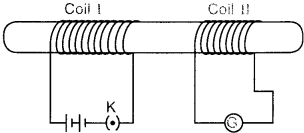(ii)When the current is passed continuously through coil I.
(iii)Name and state the phenomenon responsible for the above observation.
(iv) Write the name of the rule that is used to determine the direction of current produced in the phenomenon.
(v) Name the two coils used in this experiment.